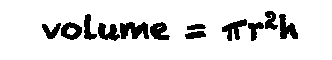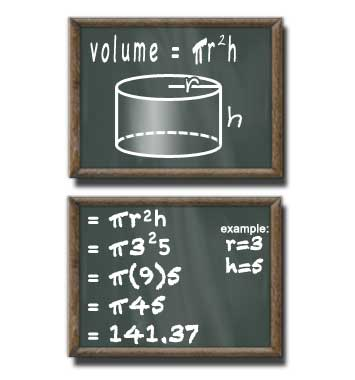# Volume of a Cylinder Calculator

## Cylinder Volume

#### For Finding Volume of a Cylinder with Circular Base

Result:
141.372height (h) decimals

A cylinder with a radius of 3 units and a height of 5 units has a volume of 141.372 cubed units.

This calculator and more easy to use calculators waiting at www.KylesCalculators.com### Calculating the Volume of a Cylinder:

If a cylinder has a flat bottom, meaning the height and radius meet at right angles, then this formula can be used to find of volume ('V') of that cylinder:

V = PI*r2h

In plain english the volume of a cylinder can be calculated by squaring the radius, multiplying that value by PI, then multiplying by the height. You can also think of it as finding the area of a flat circle (PI * radius squared) and multiplying by the height to find volume.

Here is a step-by-step case that illustrates how to find the volume of a cylinder with a radius of 3 inches and a height of 5 inches. In this example PI will be 3.14.

• V = pi * r2 * h
• = pi * 32 * 5
• = pi * 9 * 5
• = 3.14 * 45
• = 141.37 cubic inches

### Need help converting volume to other units?

Volume unit converts